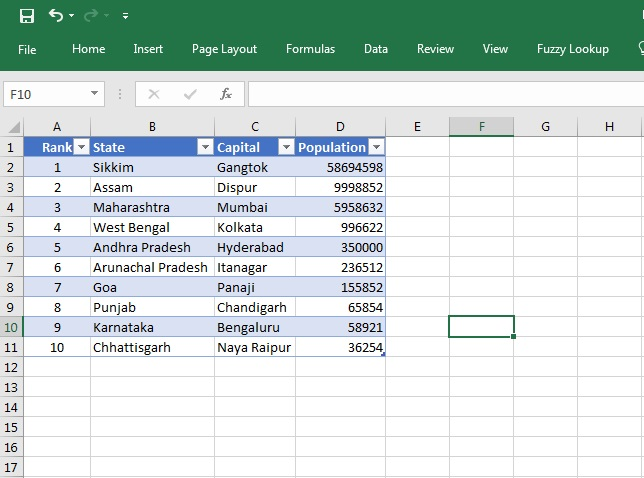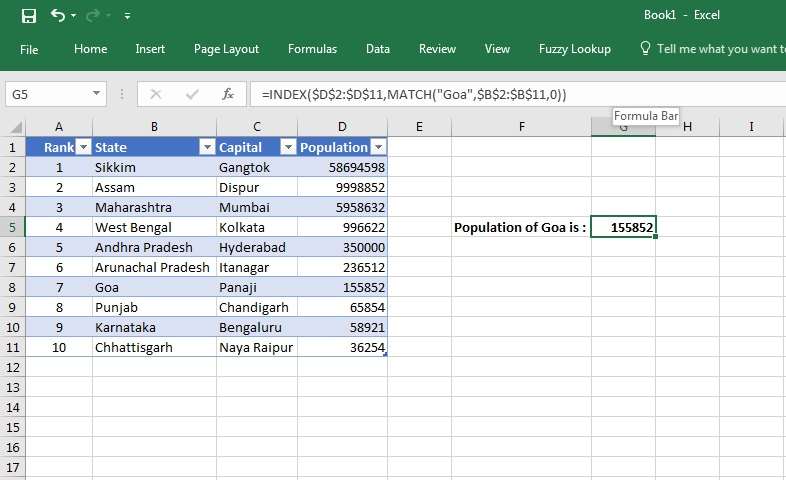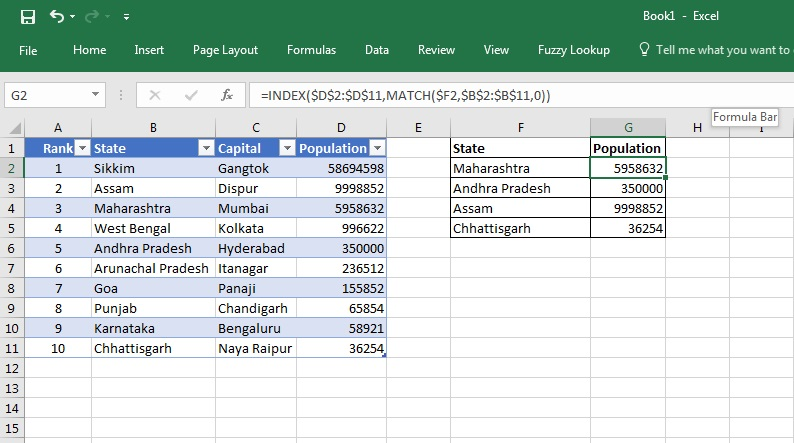How to compare two lists in Excel - KING OF EXCELKINGEXCEL.INFO ( KING OF EXCEL ) Welcome KINGEXCEL.INFO - Nothing Is Unable ... About Excel Tricks, Learning VBA Programming, Dedicated Software, Accounting, Living Skills ...

# How to compare two lists in Excel

In the tutorial, we will learn: How to compare two columns in Excel. We use various ways to compare two cells using functions in Excel. Some of these ways are:
• Comparing two columns row-by-row.
• Comparing many columns for row matches or differences.
• Highlight matches or differences between two columns.
• Highlight row matches and differences.
• Compare two lists and pull matching data.
Think sometimes we may want to match two columns in excel. Besides, we may want to pull out values for corresponding matching cells – from one table to another. In such cases, there is a combination of two functions that could help achieve the desired result.
Using INDEX and MATCH functions together makes this obtainable. Let us see what each function is and its syntax before we move to see the usage in an example.
INDEX and MATCH Functions:
• The INDEX function returns a value in an array based on the row and column numbers we specify.
• Syntax for the Index function is INDEX (array, row_number, [column_number])
• The MATCH function searches for a lookup value in a range of cells. It then returns the relative position of that value in the range.
• Syntax for the Match function is MATCH(lookup_value, lookup_array, [match type])
Note: There is also a function vLookup in Excel that does something similar. But using these formulae gives us more flexibility and control over what we want to do with our cells. Let’s use the Case Study below to show this.Step 1: Suppose we have a list of State Capitals like the ones given above along with the population of each.

Now, we want to find out the population of any particular state or a list of states from the above. We can do it using the INDEX and MATCH functions.Step 2: Now, we wish to find out the population for GOA. We can do so using the INDEX and MATCH function by entering this in the Formula Bar:
=INDEX(\$D\$2:\$D\$11,MATCH(“Goa”,\$B\$2:\$B\$11,0))
Executing this command gives us the result in cell G5. This is the correct population of Goa as per the table alongside.
There are some variations to the way the function can be used. Let us see another use of the same.Variation in Step 2: Suppose, we want to find out the population for a table of States like shown above. Then we do the following variation in the formula on the first cell where the population needs to be found:
=INDEX(\$D\$2:\$D\$11,MATCH(\$F2,\$B\$2:\$B\$11,0))
We then drag the formula across the table to get the result as above.
This formula checks the value for each entry in the array.
Knowing these functions helps in data analysis, where one has to compare data in each row.

TIP: Using \$B2:\$B11 in the formula instead of \$B:\$B makes the formula work faster. If you have a small table or one where you know the parameters of the array then give the complete table count. It will help in the calculation getting done much faster than if the full array is unspecified.
#evba #etipfree #kingexcel
📤You download App EVBA.info installed directly on the latest phone here : https://www.evba.info/p/app-evbainfo-setting-for-your-phone.html?m=1